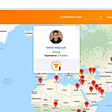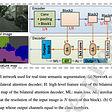# Decision Tree — My Interpretation

While making decisions we tend to assume lots of if-buts scenarios and then come up to a conclusion. Decision tree in machine learning also work in the similar concept. Visually, it is a flowchart like structure, it consist of : parent node , branch nodes and leaf nodes.

Referring to the above image Figure 1.0, Age is the parent node, Eat Pizza & Exercises are the branch nodes, Fit & Unfit are the leaf nodes. What it tells is that if your age is less than 30 and you eat pizza you are unfit. Such chain of if and else forms a decision tree.

# Decision Tree in ML

What we have is a historical data, when we apply decision tree over it, decision tree divides data into smaller and smaller chunks to create tree. Decision tree applies a function on the data to divide the data. Based on the function, data falls under segregated segment or bucket of label class for eg: Yes & No. When data is divided into smaller chunks or buckets, the target column become more homogeneous. Until the tree reaches the perfect homogeneous buckets, it continues to divide the tree further. As we divide tree, number of buckets(branches) increases.

Homogeneous bucket means a bucket consisting all same labels

The relationship between independent variable(s) and dependent variable is being expressed by function used to divide tree. This means Decision Tree is non parametric algorithm. Which means that the model does not return the model parameters. Hence, data s divided into two subset at each iteration and these subsets are called branches.

When we apply decision tree on training data, the algorithm comes out with very large and complex tree, i.e it will have many branches, leafs, nodes, buckets, such that in the last bucket your target column is perfectly homogeneous. However you may find only single record in each leaf node. Such trees are called overfit trees and hence we need to regularize tree.

Regularization means controlling growth of the tree when it is trained.

When decision tree becomes very large, they overfit. We regularize such trees, i.e trees does not grow to its full possible potential, it gets restricted. Hence, you might end up with leaf nodes where target is not perfectly homogeneous. Hence we calculate probability of each class in the bucket. Test record belongs to that class whose probability in the bucket is high. This probability is called posterior probability.

There is a problem with Decision Tree that we miss out on some combinations of data points, which is either not part of training data or being left out. Hence decision tree does miss-classification.

Steps of building decision tree :

• In decision tree, original dataset represents root node.
• Root node is broken into two buckets, these buckets are called Branch Nodes, after applying some function over root node.
• Unless regularization, when branch node have homogeneous targets, they are not further split. These last nodes are called leaf nodes.

Now, question arises how to decide on which column and its threshold the decision tree should split node to its branches and decide the threshold the decision tree should split node to its branches ?

• Decision tree uses learning mechanism called loss function. Loss function represents a way of reducing the impurity in the target column.
• To calculate impurity in any particular node we use :
a) Entropy
b) Gini
• More heterogeneous your leaf are, more uncertainty will be there, i.e high probability of misclassification.

a)Entropy :

Entropy is relation between probability and impurity. On X-axis you have Probability and on Y-axis you have impurity. You can see at Probability = 0.5 you have maximum uncertainty.

The above image shows the formula of entropy. log2 pi has following properties :

• log2(pi=1) = 0
• log2(pi=.5) = 1
• log2(pi=0) = infinity,

Hence when pi=0, the entropy curve would move towards infinity, but range of entropy is between 0–1, hence we multiply pi with log2(pi) so that the curve instead of going to infinity, it ends up at 0.

Entropy states :

• Whenever the probability of an event, i.e P(X=1) is .5, there is maximum uncertainty.
• When probability of an event is 0 or 1, uncertainty is 0.

Reason for negative sign in formula is that log2(pi) returns negative number.

Entropy in relevance to Decision Tree :

• Decision tree finds an independent attribute & within the attribute it will also find a threshold value such that when algorithm applies function on the given column, on given threshold, it breaks the data into two nodes.
• When sub branches are created, the total entropy of sub-branches should be less than the entropy of parent node. More the drop, more the information gained. Hence to split, such column is selected which gives maximum drop in entropy.
Information Gain = Entropy Previous node-Entropy Current Node

b) Gini

• There won’t be huge difference in output by choosing any of the two : entropy and gini.

Above figure demonstrate Gini index formula.

# Information Gain

• H(X) : It is entropy for root node and each split node
• |Xv| : Number of samples in branch node
• |X| : Number of samples in total
• H(Xv) : Entropy of branch node
• G(X,A) : Information Gain

H(F1) = -[(9/14 )*log2(9/14) + (5/14)*log2(5/14)]=.91

H(F2)=-[(6/8)*log2(6/8) + (2/8)*log2(2/8)]=.81

H(F3) = -[(3/6)*log2(3/6) + (3/6)*log2(3/6)]=.1

Gain=.91 - [[(8/14)*.81]-[(6/14)*.1]] = .0049

Number of records in the node decide how much noise they are contributing to overall data.

# Key Takeaway:

• Setting entropy as criterion will slow the computation because of log operation
• With splitter set as ‘random’ use hyper-parameters like : max_features, random_state. With this there are chance of using non-important column which does note give much information. Which leads to more deeper and less precise tree. But it is fast, less prone to overfitting because of randomness and you are not calculating best split before each split
• When you’ve few features you use ‘best’ as splitter
• In case max_depth is set as default, leaf node will have only one label, if you choose default value for min_samples_leaf
• If you specify min_samples_split, node will expand until all leaves contains less than minimum number of samples in comparison to max_depth. Algorithm will choose hyper-parameter which gives maximum depth over other.
• Deeper you allow your tree to grow, more complex model becomes because you’ll have more splits and it capture more information about training data. Due to this over fitting happens
• If your model overfits, reduce max_depth
• Let your model decide max_depth by default, based on the score on train and validation set increase or decrease the max_depth
• Ideal value for min_samples_split : 1 to 40. It controls overfitting. Higher value prevents model to learn relations.
• In case of imbalanced class define min_weight_fraction_leaf hyper-parameter. Give higher weights to imbalanced class.

# Things to Note

Tree complexity is measured via :

• Number of nodes
• Number of leaves
• Depth
• Number of attributes

Stopping Criteria :

• max_depth
• min_samples_split
• min_samples_leaf

I hope this content help you clear your concepts regarding decision tree. If it helps you, kindly share with your peers and grow the chain.

--

--

--

## More from Analytics Vidhya

Analytics Vidhya is a community of Analytics and Data Science professionals. We are building the next-gen data science ecosystem https://www.analyticsvidhya.com

## This Is What You Need to Know to Serve a Machine Learning Model## UNET for Semantic Segmentation — Implementation from Scratch## Guide to passing the TensorFlow Developer Certification Exam## Pytorch performance tuning in action## The 3 Basics of Machine Learning (No Math)## Universal Trading for Order Execution with Reinforcement Learning## Evaluating classification models simplified.## REGRESSION ANALYSIS## How Ensemble Technique Helps Random Forest To Achieve Higher Accuracy